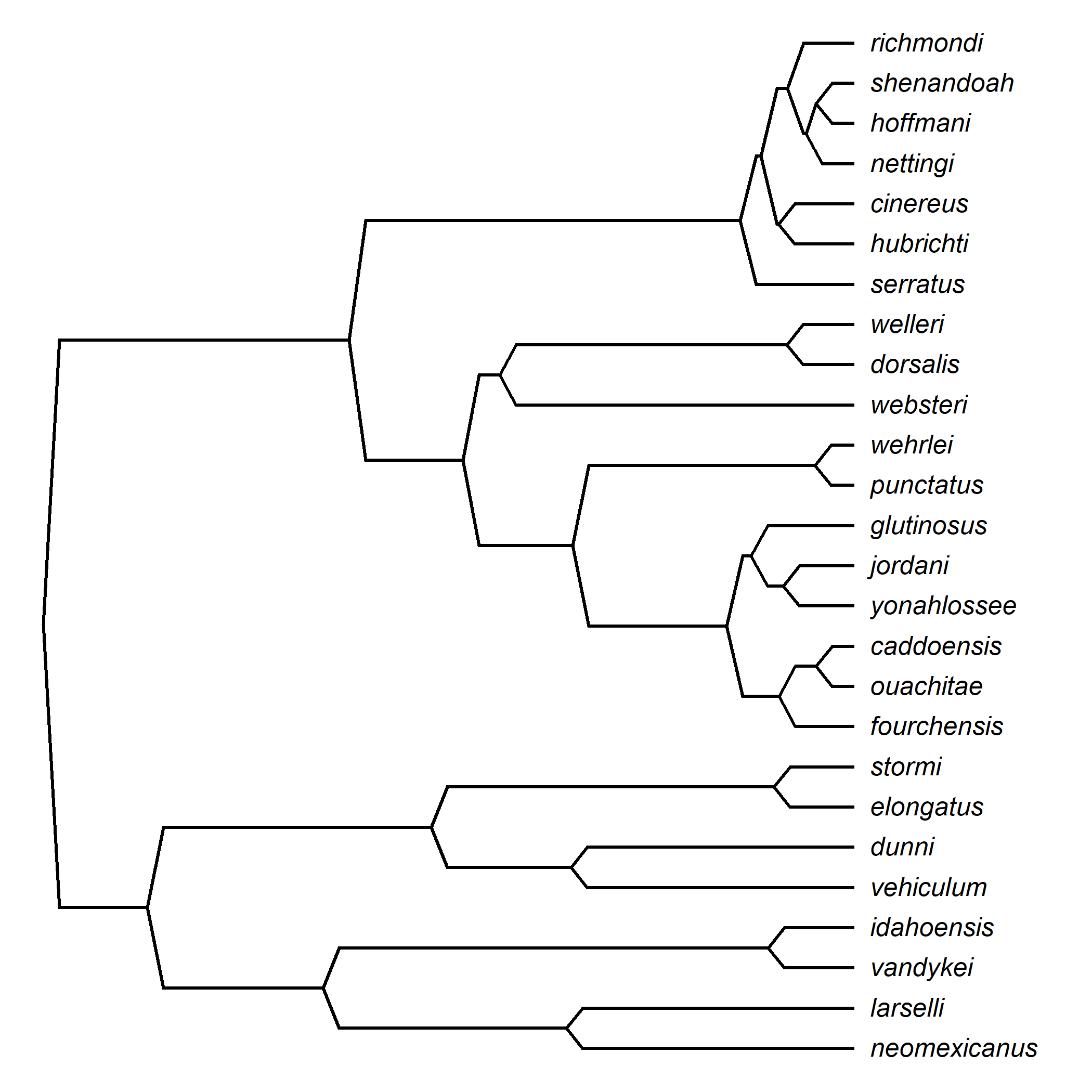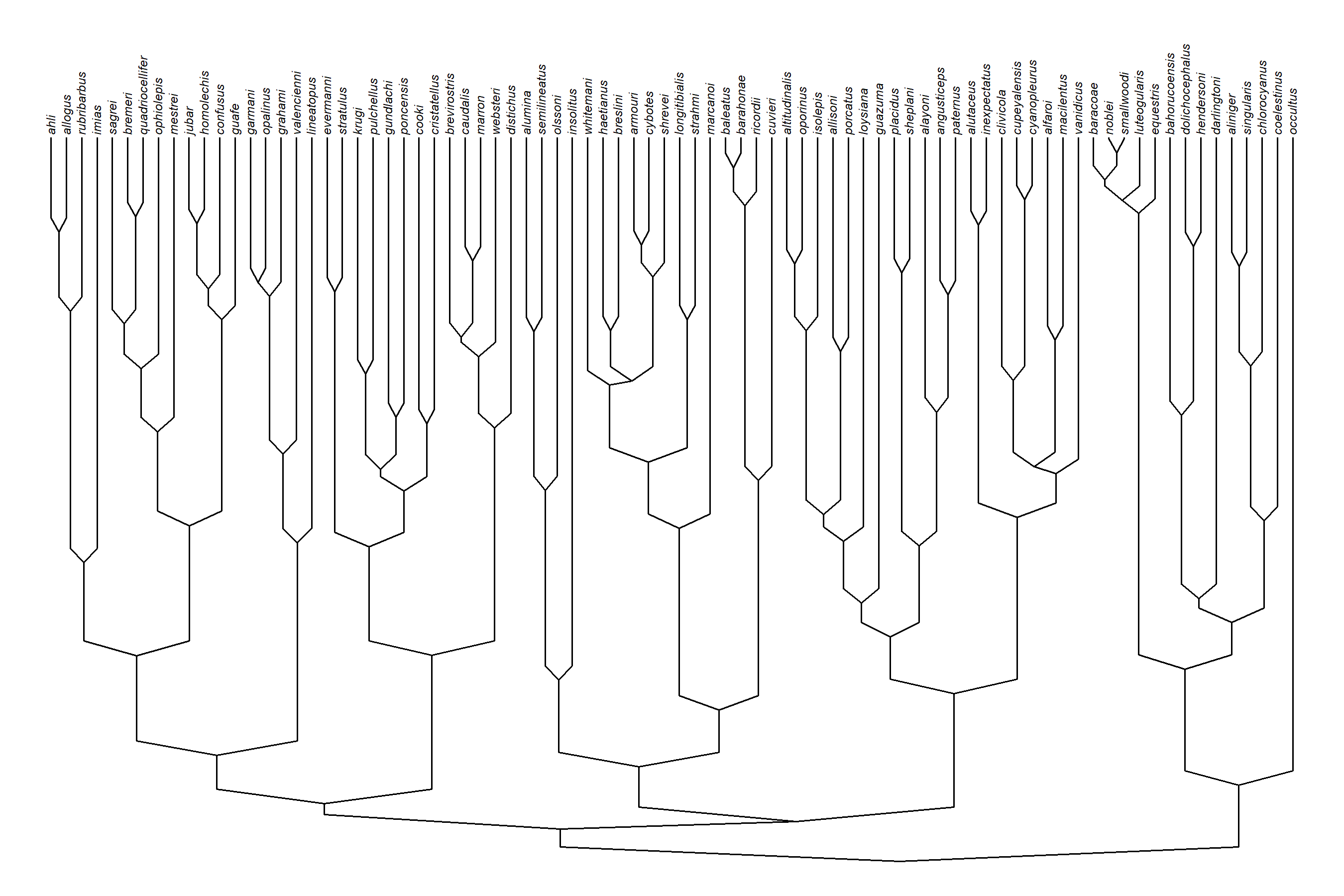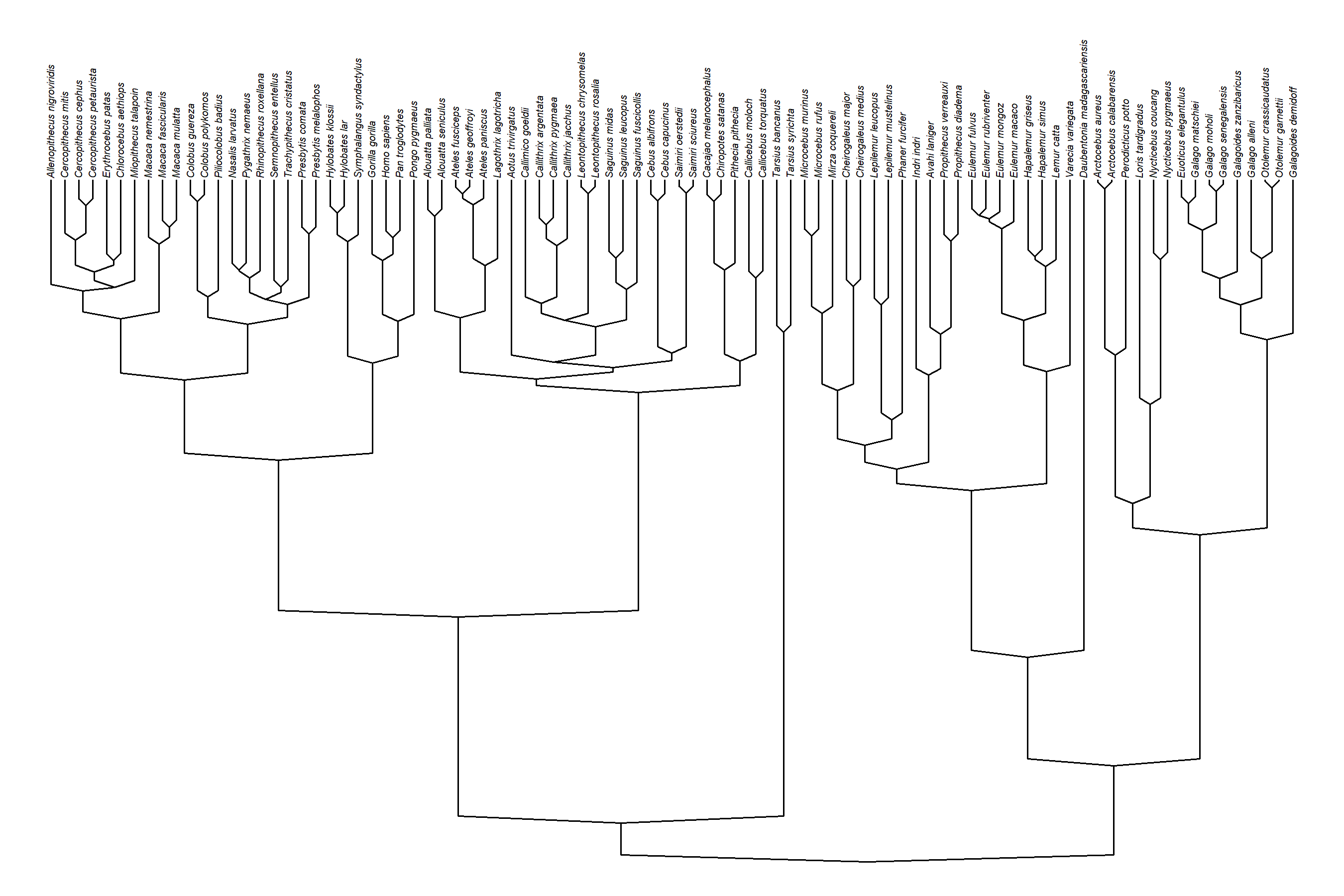## Sunday, November 12, 2023

### Creating custom phylogeny plotting styles using phytools in R

The theme of the last chapter of my recent book was empowering the reader to be creative about plotting phylogenies & phylogenetic data in R, by helping them to better understand the algorithms of tree plotting methods. There’s even a small section on writing custom plotting methods in R language.

In that vein, I stumbled today on a phylogeny plotting style that (as far as I know) has never been programmed for R. It’s harder to explain than it is to show, so better that I do the former than attempt the latter.

For the demo, I’m going to use a phylogeny of salamanders from Highton & Larson (1979).

``````library(phytools)
data(salamanders)
## start by computing coordinates for our plot
plotTree(salamanders,plot=FALSE)
pp<-get("last_plot.phylo",envir=.PlotPhyloEnv)
## calculate 2% of the total tree height
h<-0.02*(max(pp\$xx)-min(pp\$xx))
## set plotting parameters
par(lwd=2,ljoin=1,lend=1)
## plot the edges of our tree
for(i in 1:nrow(salamanders\$edge)){
edge<-salamanders\$edge[i,]
elength<-salamanders\$edge.length[i]
if(elength<h) lines(pp\$xx[edge],pp\$yy[edge])
else {
lines(pp\$xx[edge[c(1,1,2)]]+c(0,h,0),
pp\$yy[edge[c(1,2,2)]])
}
}
text(pp\$xx[1:Ntip(salamanders)],
pp\$yy[1:Ntip(salamanders)],
salamanders\$tip.label,pos=4,font=3)
``````Neat. Now let’s turn it into a function in which we can plot the tree either rightwards or upwards, and in which the height of the “slanty bit” of each plotted edge can be controlled by the user.

``````slantedTree<-function(phy,h=0.02,direction=c("rightwards","upwards")){
direction<-direction
plotTree(phy,plot=FALSE,direction=direction)
pp<-get("last_plot.phylo",envir=.PlotPhyloEnv)
h<-h*max(nodeHeights(phy))
par(ljoin=2,lend=1)
for(i in 1:nrow(phy\$edge)){
edge<-phy\$edge[i,]
elength<-phy\$edge.length[i]
if(elength<h) lines(pp\$xx[edge],pp\$yy[edge])
else {
if(direction=="rightwards"){
lines(pp\$xx[edge[c(1,1,2)]]+c(0,h,0),
pp\$yy[edge[c(1,2,2)]])
} else if(direction=="upwards"){
lines(pp\$xx[edge[c(1,2,2)]],
pp\$yy[edge[c(1,1,2)]]+c(0,h,0))
}
}
}
text(pp\$xx[1:Ntip(phy)]+
if(direction=="rightwards") 0.5*strwidth("o")*par()\$cex else 0,
pp\$yy[1:Ntip(phy)]+
if(direction=="upwards") 0.5*strheight("o")*par()\$cex else 0,
gsub("_"," ",phy\$tip.label),pos=4,offset=0,
srt=if(direction=="upwards") 90 else 0)
}
``````

OK. So, now we should be ready to try it out.

``````data(anoletree)
par(cex=0.5,font=3)
slantedTree(anoletree,direction="upwards")
````````````data(primate.tree)
par(cex=0.4,font=3)
slantedTree(primate.tree,direction="upwards",h=0.01)
``````That’s it.